Next: Arbitrary velocity structures Up: THE GENERALIZED PHASE-SHIFT METHOD Previous: THE GENERALIZED PHASE-SHIFT METHOD

## Horizontally Uniform Structures

The 2Nx by 2Nx matrix A can be partioned according to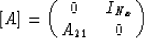(5)
where INx denotes the Nx by Nx identity matrix and the Nx by Nx submatrix A21 is given by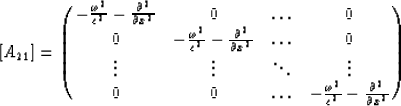(6)
The horizontal discretization requires an approximation for the derivative term in equation (6). As with the ordinary phase-shift method (Gazdag, 1978), this derivative can be calculated by Fourier method(7)
Since multiplication in the space domain is equivalent to convolution in the wavenumber domain, the multiplication of the sloth (squared slowness) becomes convolution. For horizontally uniform structures, however, the Fourier transform of the velocity function is a delta function. Therefore, the submatrix A21 has the form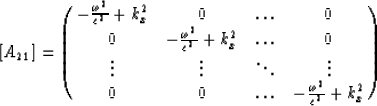(8)
The diagonal elements of this matrix are the eigenvalues of A21 and a matrix in which the columns consist of the eigenvectors form an identity matrix. By letting the eigenvalues of submatrix A21 as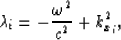the eigenvalues of matrix A become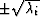, for i=1,...,Nx-1. The corresponding eigenvectors are given by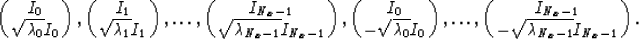where Ii denotes i-th column of identity matrix. With these eigenvectors we define a 2Nx by 2Nx matrix Q in which the columns consist of the eigenvectors of A. We then have the relation(9)
whereis the diagonal matrix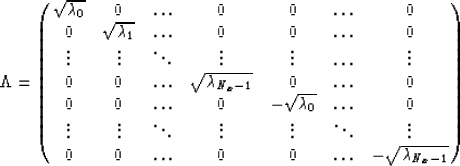(10)
and(11)
When both sides of equation (9) are multiplied by Q-1 from the left we obtain(12)
The equation (12) is a matrix representation for a set of equations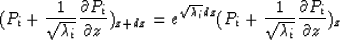(13)
and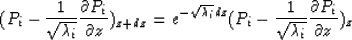(14)
The solutions of equations (13) and (14) represent upgoing and downgoing waves respectively. When an eigenvalueis real, the component is propagated by a multiplication by a real exponential, and results in an evanescent component which should be removed for numerical stability. When an eigenvalueis imaginary, the corresponding coefficient is propagated by phase shift.Next: Arbitrary velocity structures Up: THE GENERALIZED PHASE-SHIFT METHOD Previous: THE GENERALIZED PHASE-SHIFT METHOD
Stanford Exploration Project
1/13/1998